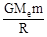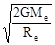# Gravitation

#### Escape Velocity

(a) Escape velocity is the minimum velocity that should be given to the body to enable it to escape away form the gravitational field of earth.
(b) The energy given to the body to project it with the escape velocity is called the 'Escape Energy' or 'Binding Energy'.
(c) Total energy of a body is reduced to zero to enable it to escape away from the gravitational field of earth.
(d) The gravitational potential energy of a particle at the surface of earth. = -Escape Energy or Binding Energy = +if thrown with the velocity ve, thenmve2=Escape velocity for earth ve == 11.2 km/sec.
(e) The value of escape velocity does not depend upon the mass of the projected body, instead it depends on the mass and radius of the planet from which it is being projected.
(f) There is no atmosphere on the planets where the root-mean square velocities more than the escapevelocity.
(g) The value of escape velocity does not depend on the angle and direction of projection instead it depends on density, mass and acceleration due to gravity of the planet.

If you want to give information about online courses to other students, then share it with more and more on Facebook, Twitter, Google Plus. The more the shares will be, the more students will benefit. The share buttons are given below for your convenience.

#### IIT (Class X)

• Unit, Dimension & Error
• Vectors
• Motion in One Dimension
• PROJECTILE MOTION
• NEWTON'S LAWS OF MOTION & FRICTION
• WORK, POWER, ENERGY & CONSERVATION LAWS
• CIRCULAR MOTION & ROTATIONAL DYNAMICS
• GRAVITATION